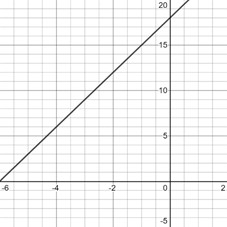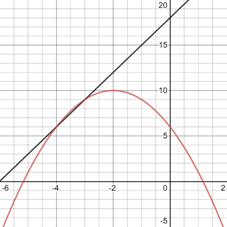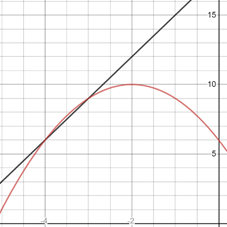# To graph : The equations y = 6 − 4 x − x 2 , y = 3 x + 18 in the viewing rectangle [ − 6 , 2 ] by [ − 5 , 20 ] and identify the intersection points.### Precalculus: Mathematics for Calcu...

6th Edition
Stewart + 5 others
Publisher: Cengage Learning
ISBN: 9780840068071### Precalculus: Mathematics for Calcu...

6th Edition
Stewart + 5 others
Publisher: Cengage Learning
ISBN: 9780840068071

#### Solutions

Chapter 1.9, Problem 25E
To determine

## To graph: The equations y=6−4x−x2,y=3x+18 in the viewing rectangle [−6,2]by[−5,20] and identify the intersection points.

Expert Solution

### Explanation of Solution

Given information:

The equations y=64xx2,y=3x+18 and viewing rectangle [6,2]by[5,20] .

Graph:

The graph of the equation y=64xx2 can be sketched using the table,

 x y 0 6 2 −6 4 26

The graph of equation is provided below,The graph of the equation y=3x+18 can be sketched using the table,

 x y 0 18 2 24 −2 12 −1 15

The graph of equation is provided below,The graph of both equations in same figure is provided below,From the graph it looks as the graphs intersect near the (3,8) .Zoom the area near this point.Since, the graphs intersect each other at 2 points.

Thus, the graphs intersect in the given viewing rectangle. There are 2 intersection points.

Interpretation:

The graph of an equation in a viewing screen is a viewing rectangle.

The x -values to range from a minimum value of xmin =a to a maximum value of xmax =b

The y -values to range from minimum value of ymin =c to a maximum value of ymax =d

Then, the display portion of the graph lies in the rectangle [a,b]×[c,d]={(x,y)|axb,cyd}

The equations y=64xx2,y=3x+18 are intersect at 2 points in viewing rectangle [6,2]by[5,20] .

### Have a homework question?

Subscribe to bartleby learn! Ask subject matter experts 30 homework questions each month. Plus, you’ll have access to millions of step-by-step textbook answers!